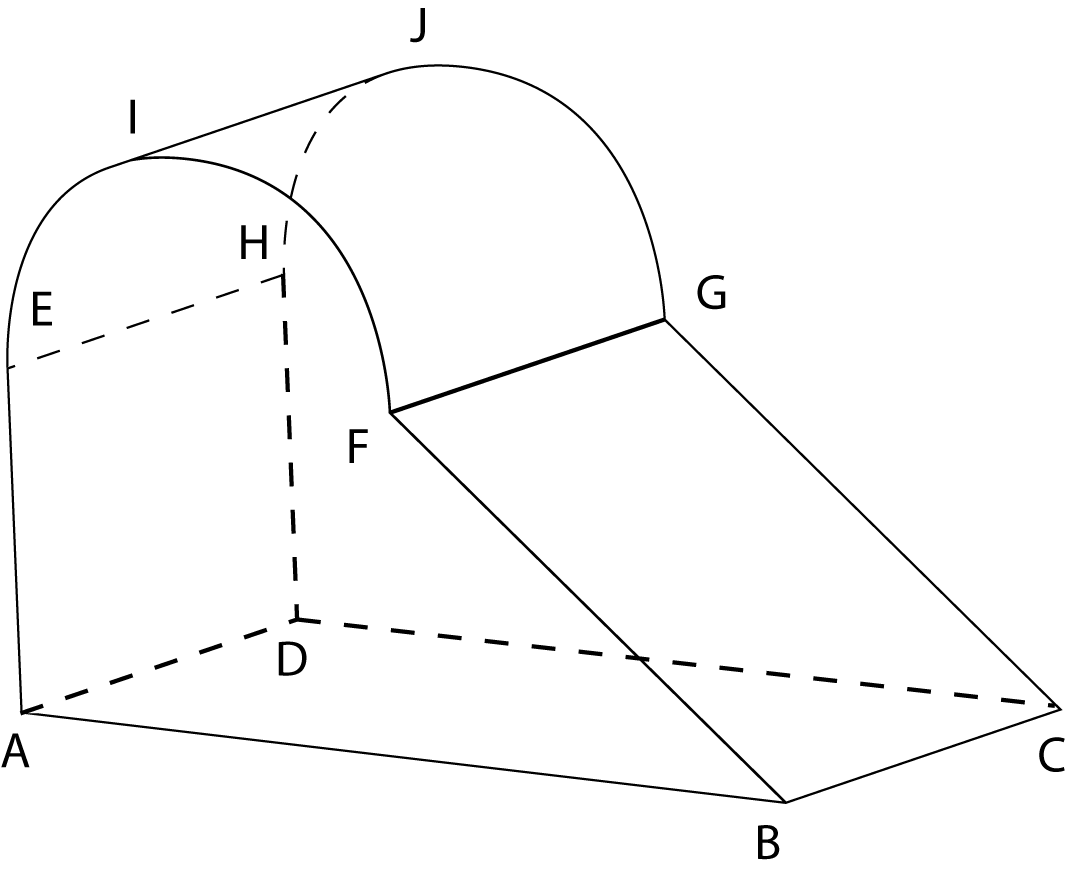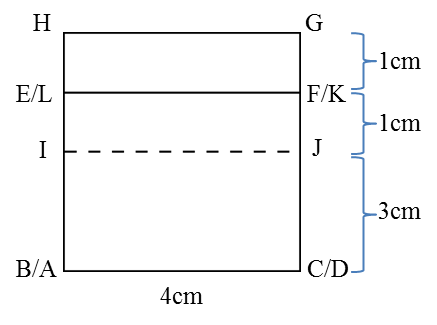# 10.3.1 Plan and Elevation, SPM Paper 2 (Long Questions)

Question 1:The diagram above shows a solid consisting of a right prism and a half-cylinder which are joined at the plane EFGH. EF is the diameter of the semi-circle and is equal to 3 cm.

The base ABCD is on a horizontal plane and AB = 6 cm, BC = 4 cm. The vertical plane ABFE is the uniform cross-section of the prism.

Draw to full scale, the plan of the solid.

Solution:Question 2:Diagram above shows a solid right prism with rectangular base ABCD on a horizontal table. The vertical plane ABEHIL is the uniform cross-section of the prism. Rectangle LIJK, IHGJ and HEFG are inclined planes. AL, DK, BE and CF are vertical edges.

Given BC
= 4 cm, AB = 6cm. EB = FC = LA = KD = 4cm, The vertical height of  I and J from the rectangular base ABCD = 3cm, while the vertical height of H and G from the rectangular base ABCD = 5cm.

Draw to full scale, the elevation of the solid on a vertical plane parallel to BC as viewed from X.

Solution: